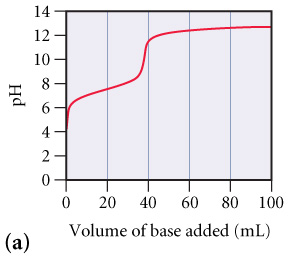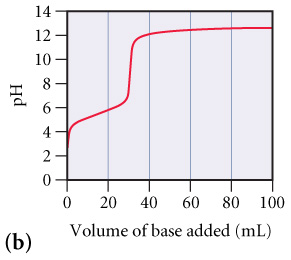# Problem: Consider the following titration curves for two weak acids (see the figures), both titrated with 0.100 M NaOH., Which of the two acid solutions is more concentrated?

###### FREE Expert Solution

Weak acid and strong base titration

• pH gradually increases as it approaches the equivalence point
• pH at the equivalence point is more than 7.00

The steepest point in the curve will be the equivalence point.

• This is basically the turning point in the curve.
• This is usually the middle point of the horizontal segment.

88% (19 ratings)###### Problem Details

Consider the following titration curves for two weak acids (see the figures), both titrated with 0.100 M NaOH.,Which of the two acid solutions is more concentrated?

Frequently Asked Questions

What scientific concept do you need to know in order to solve this problem?

Our tutors have indicated that to solve this problem you will need to apply the Weak Acid Strong Base Titrations concept. You can view video lessons to learn Weak Acid Strong Base Titrations. Or if you need more Weak Acid Strong Base Titrations practice, you can also practice Weak Acid Strong Base Titrations practice problems.